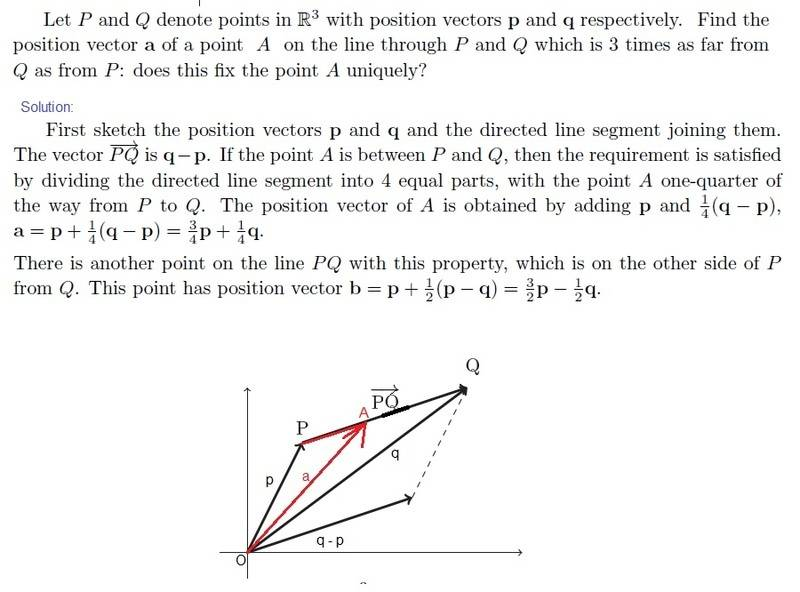# Linear Algebra QuestionPlease help me to understand the solution :( (I have made the sketch, is it correct?, where has the 1/4 comes from? is it like 1:3 (1 ratio 3) => numerator divided by numerator + denominator = 1/1+3 =1/4 (as given on page 6 at http://www.lowndes.k12.ga.us/view/14167.pdf [Broken] )? or am I wrong? For point position vector b in the solution the point B is on the left side from P (as A is on the right side of P)?)

Last edited by a moderator:

Mark44
MentorPlease help me to understand the solution :( (I have made the sketch, is it correct?, where has the 1/4 comes from? is it like 1:3 (1 ratio 3) => numerator divided by numerator + denominator = 1/1+3 =1/4 (as given on page 6 at http://www.lowndes.k12.ga.us/view/14167.pdf [Broken] )? or am I wrong? For point position vector b in the solution the point B is on the left side from P (as A is on the right side of P)?)

Your sketch isn't very accurate. It looks like A is more than 1/3 of the way along the segment between P and Q. From the problem description, the length of AQ is three times the length of AP, so A should be 1/4 of the way from P to Q.

woundedtiger4 said:
For point position vector b in the solution the point B is on the left side from P (as A is on the right side of P)?)
I don't understand what you're asking here. What point B?

Last edited by a moderator:
Mentallic
Homework Helper
where has the 1/4 comes from? is it like 1:3 (1 ratio 3) => numerator divided by numerator + denominator = 1/1+3 =1/4 (as given on page 6 at http://www.lowndes.k12.ga.us/view/14167.pdf [Broken] )? or am I wrong?

It is 1/(1+3) = 1/4. If it were 1/3, then A would be a distance of x from P while A will be a distance of 2x from Q. Can you see this? This would make the ratio 1:2 as opposed to 1:3.

For point position vector b in the solution the point B is on the left side from P (as A is on the right side of P)?)
Yes, except that the question doesn't introduce a new point B, it just asked whether A is uniquely determinable, which is no because it can be found on the left side of P as well. A has two possible positions.

Last edited by a moderator:
Fredrik
Staff Emeritus